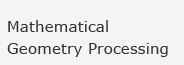Home » Shapes Explorer

## Shapes Explorer

This example uses geometries created by Mathematica's "Shapes" package.
 Cylinder [r, h ,n] cylinder with radius r, and half height h drawn using n polygons Torus [r1, r2, n, m] torus with radii r1 and r2 drawn using a n by m mesh Sphere [r, n, m] sphere with radius r drawn using m(m-2)+2 polygons MoebiusStrip[r1, r2, n] Möbius strip with radii r1 and r2 drawn using 2n polygons Helix[r, h, m, n] helix with radius r, half height h and m turns drawn using an n by m mesh Cone[r, h, n] cone with radius r and half height h drawn using n polygons

Please be patient, the server needs some time to send the data.

© 2006 Freie Universität Berlin | Feedback |
Stand: 21.04.2008